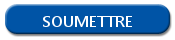# Write conditions in R: if / else

## If: execute and action if a condition is verified

To execute a. condition, you need to use the command if.

`if (myCondition){ action }`

If the condition is true (TRUE), so the action contained between the brackets is executed. Otherwise, the lines between the brackets are ignored.

The condition can be defined by several ways: by the comparison based on logical operators, or by calling a function that send a Boolean for example. Note that if you use a numeric vector, 0 is defined as FALSE.

There is an example of condition in R:

`if (variable == TRUE) { print("My instruction is executed") }`

## Else: execute an action if a condition isn't verified

With the element else, you can execute an action if the condition isn't verified. The element else must be located on the same line than the bracket that close the condition.

For example, there is a condition containing an else.

```if (variable == TRUE) { print("My condition is validated") } else {
print("My condition isn't validated") }```

Then, the conditions can be cumulated thanks to the else if elements.

```if (variable == value1) { print("My condition has the value1") } else if (variable == value2) {
print("My condition has the value 2") } else { print("My condition hasn't the valu1 or the value2") }```

## The ifelse() function

The ifelse() function can be used to simplify some condition.

`ifelse(myCondition, actionIfTrue, actionIfFalse)`